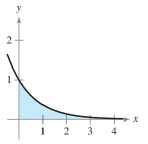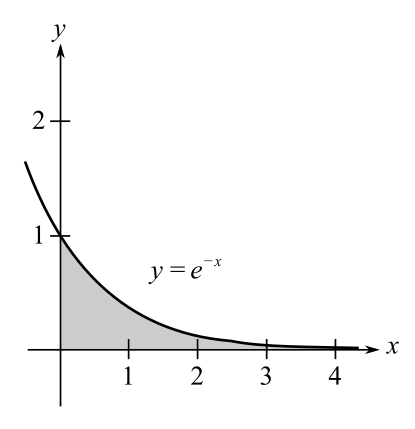Chapter 6.4, Problem 21E### Calculus: An Applied Approach (Min...

10th Edition
Ron Larson
ISBN: 9781305860919

#### Solutions

Chapter
Section### Calculus: An Applied Approach (Min...

10th Edition
Ron Larson
ISBN: 9781305860919
Textbook Problem
1 views

# Area of a Region In Exercises 21-26, find the area of the unbounded shaded region. y = e − xTo determine

To calculate: The area of the unbounded shaded region for the function y=ex.Explanation

Given Information:

The provided function is, y=ex and the graph is:

Formula used:

The area between two graphs:

If f(x)g(x) for all x in [a,b] (so that the graph of f doesn’t move below that of g), then the area of the region between the graphs of f and g and between x=a and x=b is given by:

A=ab[f(x)g(x)dx]

From definition of improper integral.

af(x)dx=limbabf(x)dx

Also, the expression for the integration of an exponential is as follows:

eaxdx=eaxa+C; where a0.

Calculation:

Here, the two functions are f(x)=ex and g(x)=0 and the graph is going from 0 to

### Still sussing out bartleby?

Check out a sample textbook solution.

See a sample solution

#### The Solution to Your Study Problems

Bartleby provides explanations to thousands of textbook problems written by our experts, many with advanced degrees!

Get Started

#### Find an equation of the line that passes through (1, 2) and (4, 5).

Applied Calculus for the Managerial, Life, and Social Sciences: A Brief Approach

#### In problems 9-18, simplify the expressions with all exponents positive. 16.

Mathematical Applications for the Management, Life, and Social Sciences

#### True or False: converges.

Study Guide for Stewart's Single Variable Calculus: Early Transcendentals, 8th

#### How does a full-text database differ from other databases?

Research Methods for the Behavioral Sciences (MindTap Course List)